# 数据结构与算法 - 基础``对自然数从1到100的求和``

``void addNum () {    int total = 0;    for (int i = 1; i <= 100; i ++) {        total += i;    }    printf("total is %d \n",total);// 5050}``

`1+2+3+....+n = n*(n+1)/2`

## 数据``struct Teacher {    char *name;    char *sex;    int age;};int main(int argc, const char * argv[]) {    struct Teacher t1;          // 数据元素    struct Teacher tArray;  // 数据对象（ 一组数据元素组成 ）    t1.name = "Mary";           // 数据项    t1.sex = "female";          // 数据项    t1.age = 23;                // 数据项    return 0;}``

## 结构

### 逻辑结构

• 集合结构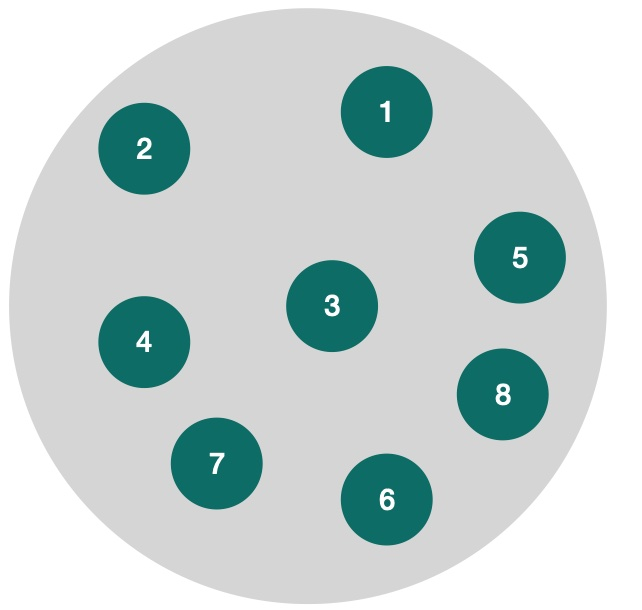集合结构中的数据元素除了同属一个集合外，彼此之间没有其他关系。

• 线性结构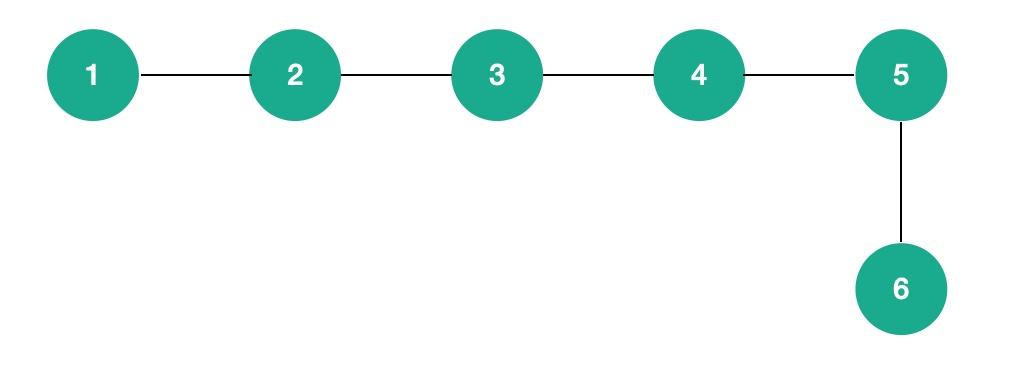线性结构中的数据元素之间都是一对一的关系，从图中也可以看出他们像一条线一样把各个元素连起来，常用的线性结构有：链表队列数组 等等。

• 树形结构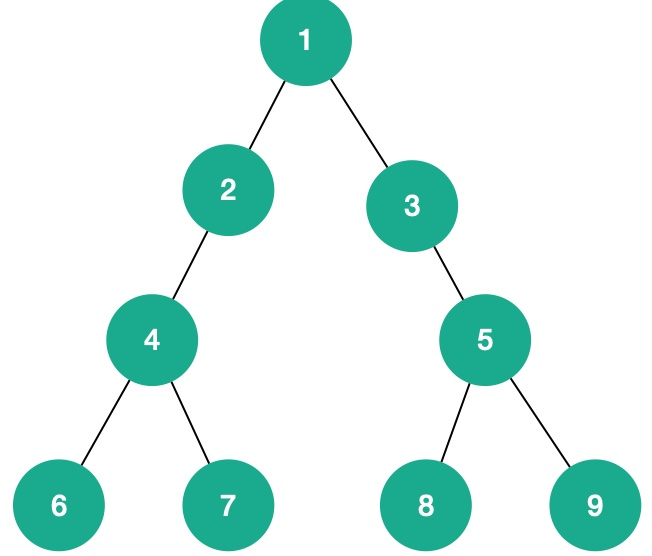树形结构中的元素是呈现一对多的关系，常见的树有：二叉树B树哈夫曼树 等等。

• 图形结构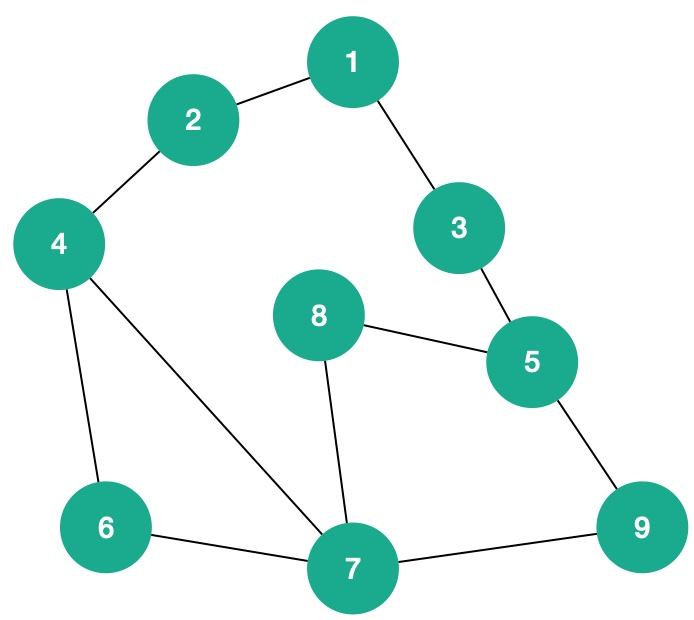图形结构之间的元素是多对多的关系，常见的图形结构有： 矩阵 等。

### 物理结构

• 顺序存储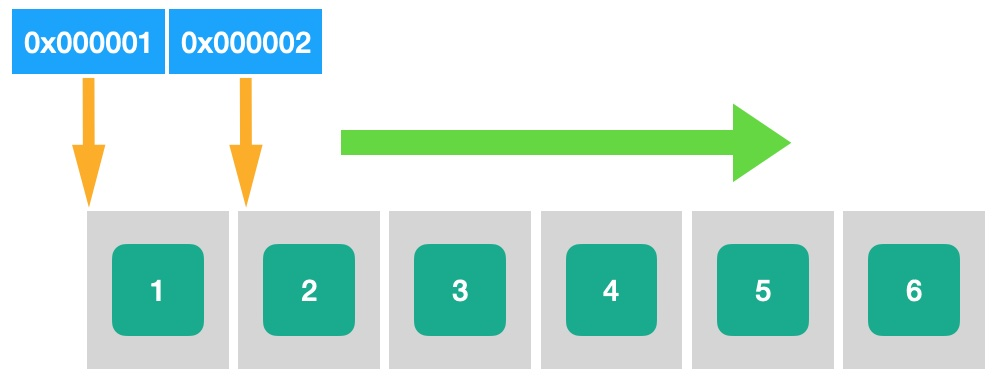我们知道计算机中的内存都是连续的，如上图所述，1-6个元素按照顺序存储的方式存到内存里，比如第一个元素的内存地址是 `0x000001` 假设我们这个顺序表中每个元素占1个位置，那么很容易得到第二个元素的地址是 `0x000002`,以此类推很容易知道第六个元素的地址是 `0x000006`

• 链式存储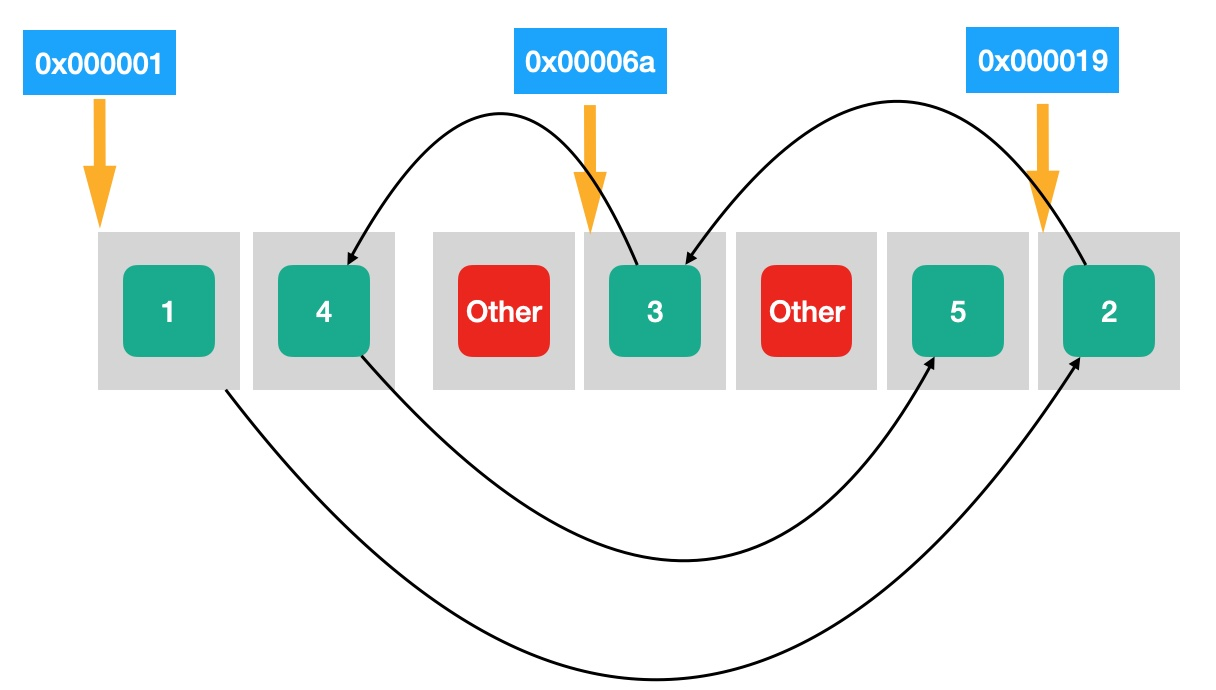这里我画了一个简单的图，大概描述了一下链式存储在内存中是如何体现的。当我们的元素在内存中是散乱的，也就是他们的地址之间没有一定的规律，这个时候就要靠我们的指针去标记位置了，比如我们把 `元素2` 的物理地址 `0x000019` 存储到 `元素1` 中去，那么每两个元素之间就会有一定的相互绑定的关系，这就是链式存储的基本逻辑。

## 算法

### 算法的特性

• 输入输出
• 有穷性 ：当然不能是无限循环了…
• 确定性 : 每一步都是明确的，不能出现模棱两可选择。
• 可行性

### 算法的设计要求

• 正确性
• 可读性
• 健壮性
• 时间效率高和存储量低 ：其实这才是算法的精髓，研究算法无非就是要提高效率和降低存储。

### 时间空间复杂度

• 用常数1取代运行时间中的所有常数
• 各种复杂度相加，只保留最高阶 `n+2n+log(n)+n^2` —–> `n^2`
• 如果最高阶存在且不是1，则去掉这个项相乘的常数 `O(5logn) -----> O(logn)`

#### 常数阶

``//1+1+1 = 3void testSum1(int n){    int sum = 0;                //执行1次    sum = (1+n)*n/2;            //执行1次    printf("testSum2:%d\n",sum);//执行1次}``

#### 线性阶

``void add2(int x,int n){    for (int i = 0; i < n; i++) {        x = x+1;    }}``

#### 对数阶

``int count = 1;while(count < n){    count = count * 2;}``

#### 平方阶

``// x=x+1; 执行n*n次void add3(int x,int n){    for (int i = 0; i< n; i++) {        for (int j = 0; j < n ; j++) {            x=x+1;        }    }}``

`O(1) < O(logn) < O(n) < O(nlogn) < O(n^2) < O(n^3) < O(2^n) < O(n!) < O(n^n)`

### 空间复杂度

``int n = 5;    int a = {1,2,3,4,5,6,7,8,9,10};    //算法实现(1)    /*    算法(1),仅仅通过借助一个临时变量temp,与问题规模n大小无关,所以其空间复杂度为O(1);    */    int temp;    for(int i = 0; i < n/2 ; i++){        temp = a[i];        a[i] = a[n-i-1];        a[n-i-1] = temp;    }    for(int i = 0;i < 10;i++)    {        printf("%d\n",a[i]);    }    //算法实现(2)    /*     算法(2),借助一个大小为n的辅助数组b,所以其空间复杂度为O(n).    */    int b = {0};    for(int i = 0; i < n;i++){        b[i] = a[n-i-1];    }    for(int i = 0; i < n; i++){        a[i] = b[i];    }    for(int i = 0;i < 10;i++)    {        printf("%d\n",a[i]);    }``

------------- 本文结束  感谢您的阅读 -------------